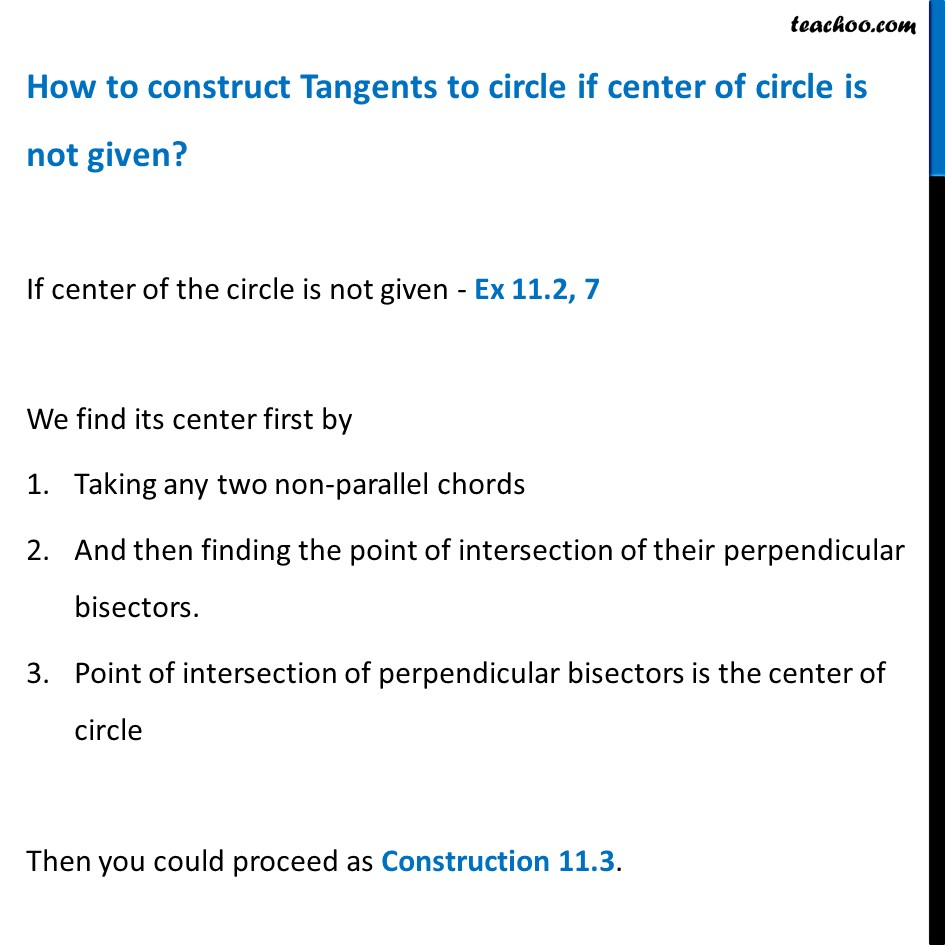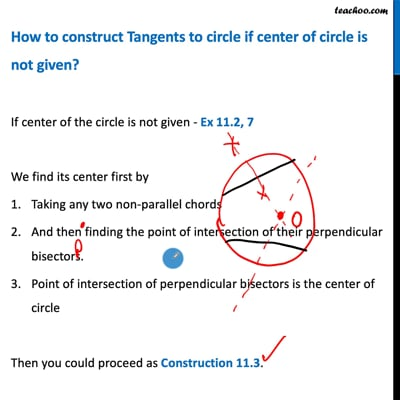Examples

Chapter 11 Class 10 Constructions (Term 2)
Serial order wiseHow to construct Tangents to circle if center of circle is not given?

If center of the circle is not given - Ex 11.2, 7

We find its center first by

1. Taking any two non-parallel chords
2. And then finding the point of intersection of their perpendicular bisectors.
3. Point of intersection of perpendicular bisectors is the center of circle

Then you could proceed as Construction 11.3 .This video is only available for Teachoo black users

### Transcript

How to construct Tangents to circle if center of circle is not given? If center of the circle is not given - Ex 11.2, 7 We find its center first by Taking any two non-parallel chords And then finding the point of intersection of their perpendicular bisectors. Point of intersection of perpendicular bisectors is the center of circle Then you could proceed as Construction 11.3.